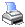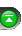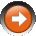﻿ Efficiency Graphs
 Operations > Control Center > Account Performance Tab > Efficiency GraphsFollowing are the formulas for the calculation of some of the efficiency performance graphs.

Assume the following:

- Enter long at price of 100

- Market moves down to a price of 90

- Market moves up to a price of 130

- Exit at a price of 110

Entry Efficiency is Calculated as:

(maximum price seen - entry price) / (maximum price seen - minimum price seen)

= (130 - 100) / (130 - 90)

= 75%

= The entry took 75% of the trade range

Exit Efficiency is Calculated as:

(exit price - minimum price seen) / (maximum price seen - minimum price seen)

= (110 - 90) / (130 - 90)

= 50%

= The exit took 50% of the available trade range

Total Efficiency is Calculated as:

(exit price - entry price) / (maximum price seen - minimum price seen)

= (110 - 100) / (130 - 90)

= 25%